# 高雄 古早味の香蕉冰＜常美冰店＞

ＮＯ。ＮＯ。ＮＯ。。我是越來越氣啦～～(早知道就開車!嗚嗚…好熱好熱,日頭赤焰焰呀!)))

(但我只想吃冰吃冰吃冰吃冰呀~~~~~~~~)))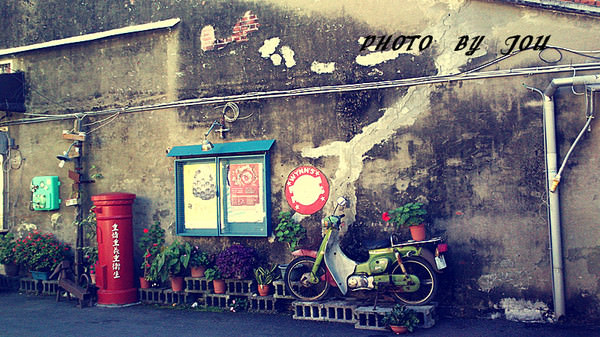感覺還蠻悠靜的小巷子，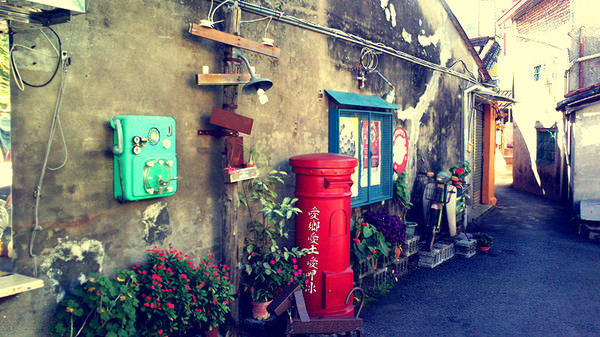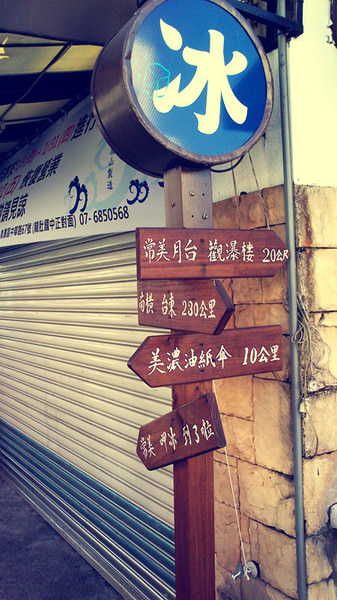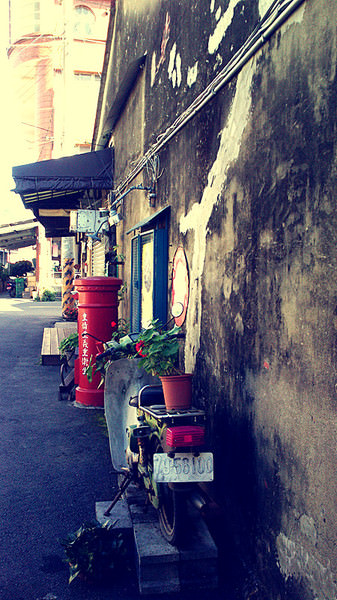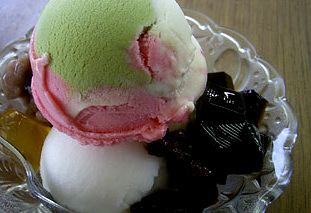可惜。來來回回走了四五十分鐘，我什麼都沒吃到～～

（再次淚奔)))))))))))))))))))))))))))))

０７—６６２７１７９